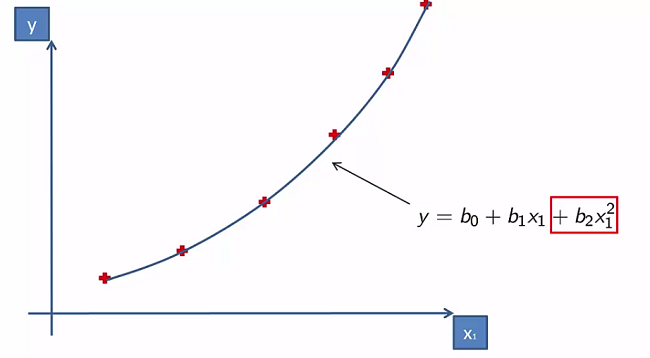## Polynomial Linear Regression

Polynomial Linear Regression Formula: $y= b_{_0} + b_{_1}X_{_1} + b_{_2}X{_1}^ + ... + b_{_n}X_{_1}^n$

Notice that there is only $X_{_1}^n$. So basically, we are using one variable but different powers of that variable.

The reason why it is still called a linear regression is because we aren’t talking about the x variable but rather the b coefficients.

Polynomial Regressions are typically used in data sets like this:## Polynomial Regression in Python

Preprocess the data with the template.

### Fitting Linear Regression to the dataset

Import the LinearRegression class from Scikit learn. Create a variable and fit the LinearRegression object to the dataset X and y.

from sklearn.linear_model import Linear Regression
lin_reg = LinearRegression()
lin_reg.fit(X, y)


### Fitting the Polynomial Regression Model to the dataset

Import the PolynomialFeatures class from scikit learn library and create an object. Fit it to the dataset.

from sklearn.preprocessing import PolynomialFeatues


When using the PolynomialFeatures class we will want to specify the argument degree to be whatever degree of the function we want. We will use the fit_transform method to fit and transform X and save it as X_poly

Note : The PolynomialFeatures class will automatically create a column of ones for the constant $b_{_0}$

poly_reg = PolynomialFeatues(degree = 2)
X_poly = poly_reg.fit_transform(X)


We now need to create a new LinearRegression object that will be fitted with X_poly and y

lin_reg_2 = LinearRegression()
lin_reg.fit(X_poly, y)